WHCSRL 技术网

# 网络安全入门：Python 基础指南## 1、Python 简介

Python是一种「基于C语言实现的」的，「开源」的，「面向对象」的，「动态数据类型」「解释型」语言。
1989年圣诞节期间，Python的「创始人吉多·范罗苏姆」（Guido van Rossum）为了打发无聊的时间，开发出了一款解释型语言，命名为Python（蟒蛇），并于1991年公开发行。

## 2、Python 的优势？

### 1）学习成本低

Python的所有内容都是免费开源的，这意味着你不需要话一分钱就可以「免费使用」Python；Python的语法简单且优雅，相对于其他语言「学习难度较低」，入门非常快。### 2）开发成本低

Python有丰富的「库」可以使用，这些库提供了大量的「基础实现」，在编码过程中，可以方便的使用这些库，从而避免了大量代码的编写过程。代码量的下降意为着「开发周期」的缩短，这在一定程度上减轻了程序员的开发负担，同时提高了开发的「效率」，正因如此，在Python领域流传着这样一句话：「人生苦短，我用Python」

### 3）语言生态丰富## 3、第一个 Python程序

### 1）新建 HelloPython.py文件### 2）编写代码

``````print('Hello Python')
```1```### 3）运行文件``````python HelloPython.txt
```1```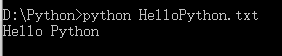### 4）修改文件扩展名``````python HelloPython.py
```1```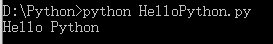## 1、注释

Python中的代码都是用「英语」写的，对于母语不是英语的我们来说，「阅读」英语的代码，肯定没有阅读「汉字」那么方便。我之前做开发的时候就深有体会，上午才写完的代码，下午再去看，就已经看不太懂了（手动捂脸）。当然，这是我自己写的代码，从头到尾捋一遍还是能明白的。那么问题来了，自己写的代码就已经这么难看懂了，要是看别人写的代码，岂不是要读天书？这个时候我们就可以使用「注释」了，注释就是对代码的「理解」「说明」，可以帮助开发者了解代码的含义

### 1）使用场景

``````# 这行代码的意思是定义一个变量
name = '张三'
```12```

### 2）语法规范

Python作为一款「优雅」的语言，对于代码的「语法格式」有着近乎「苛刻」「规范」，这其中自然也包括注释

1. Python注释通常以 # 开头
2. 注释「单独一行」时， # 「左边」需要「顶格」写，不允许有空格； # 「右边」和注释内容之间需要有一个「空格」作为「间隔」，以增加代码的「可读性」
3. 注释写在「代码后面」时， # 「左边」需要有「两个空格」 # 「右边」和注释内容之间需要有一个「空格」作为「间隔」

### 3）单行注释

``````# 单行注释
print('hello Python')
```12```

``````print('hello Python')  # 单行注释
```1```

### 4）多行注释

``````# 这段代码的作用是
# 作用一：***
# 作用二：***
print('假装是一段代码')
```1234```

### 5）三重引号字符串

``````'''

'''
print('假装是一段代码')
```123456```

``````"""

"""
print('假装是一段代码')
```123456```

## 2、Python 变量

``````# 我的存款是100块钱

# 我将50块钱工资放到存款中

# 存款发生了变化，最终的存款是
print(存款)
```12345678```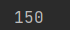### 1）语法规范

1. 变量不需要「声明」，变量的「赋值操作」即是变量声明和定义的过程
2. 变量使用等号（=）赋值，变量被赋值以后才会被「创建」，未赋值的变量不能被使用
3. 等号（=）左边是「变量名」变量名，右边是存储在变量的「值」，即 `变量名 = 变量值`
4. 定义变量时，不需要指定「变量类型」，程序运行时，Python解释器会根据等号右侧的数据自动推导出数据类型

### 2）数据类型

• Numbers（数字）
• String（字符串）
• List（列表）
• Tuple（元组）
• Dictionary（字典）

## 3、Numbers

Python中使用Numbers来表示「数字类型」，数字类型包括「整数」「小数」等多种类型，在Python中，整数用int来表示，小数用float来表示

### 1）算数运算符### 2）整数（int）

「整数」就是没有小数位（小数位都是0）的数、能被1整除的数。为了方便数据类型的学习，这里先扩展一个函数：type()，type（）函数可以用来「检查数据的类型」，并返回检查的结果。接下来我们通过几个案例来了解一下变量的使用

``````# 定义一个整数类型的变量
number = 1

# 判断变量类型
result = type(number)

# 打印变量类型
print(result)
```12345678```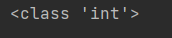``````# 苹果单价是3元
apple = 3

# 苹果的数量是2
number = 2

# 总价 = 单价 * 数量
money = apple * number
print(money)
```123456789```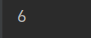### 3）浮点数（float）

``````# 定义一个小数类型的变量
number = 1.5

# 检查变量的类型
result = type(number)

# 打印变量类型
print(result)

```123456789```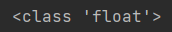``````# 苹果的价格是1.5
apple = 1.5

# 苹果的数量是3
number = 3

# 总价 = 价格 * 数量
result = apple * number

# 打印结果
print(result)
```1234567891011```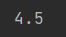## 4、String 初识

### 1）字符串的定义

``````str1 = '单引号字符串'
str2 = "双引号字符串"
```12```

### 2）字符串拼接

``````# 定义一个数字
number1 = 2

# 定义第二个数字
number2 = 3

# 输出两个数字的拼接结果
print(number1 + number2)
```12345678```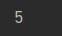``````# 定义一个字符串
str1 = "abc"

# 定义第二个字符串
str2 = "def"

# 输出两个字符串的拼接结果
print(str1 + str2)
```12345678```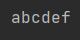``````# 定义一个字符串
str1 = "abc"

# 定义一个数字
number = 2

# 输出两个字符串的拼接结果
print(str1 + number)
```12345678```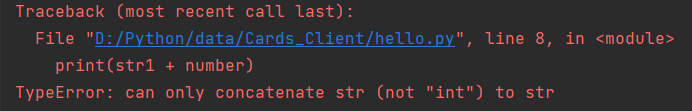``````# 定义一个字符串
str1 = '*'

# 定义一个数字
number = 10

# 打印字符串和数字的拼接结果
print(str1 * number)
```12345678```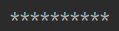## 三、Python 流程控制语句

Python中提供了三种流程控制语句

• 顺序执行
• 分支执行
• 循环执行## 1、if 条件判断语句

``````if 老人和孩子:
满足条件，做救生艇逃走
else:
不满足条件，留下来
```1234```

### 1）基本语法

``````if 判断条件:
条件成立时,要做的事情
else:
条件不成立时，要做的事情
```1234```

``````# 定义人物属性
man = '老人'

# 判断是否是老人
if man == '老人':
print('老人快跑')
else:
print('年轻人，面对困难')
```12345678```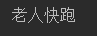### 2）if

if 条件判断语句并不是非得写成案例1的样子，最「简单」的语法格式是下面这样

``````if 条件判断:
满足条件执行的代码
```12```

``````# 定义张三的成绩
zhangsan = 100

# 判断成绩
if zhangsan >= 100:
print('棒棒糖x1')
```123456```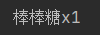### 3）if else

if else 是if 条件判断语句的「常规」格式，可以简单理解为：如果怎么样，就怎么样，否则就怎么样。接下来，我们通过案例来简单了解一下

``````# 定义年龄
age = 18

# 判断年龄
if age >= 18:
print('去网吧玩游戏')
else:
print('滚回家写作业')
```12345678```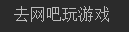### 4）if elif else

``````if 判断条件:
条件成立时,要做的事情
elif 判断条件:
if条件不成立,但elif条件成立时,要做的事情
else:
条件都不成立时,要做的事情
```123456```

``````# 定义张三的成绩
zhangsan = 100

# 判断成绩
if zhangsan >= 100:
print('女朋友x1')
elif zhangsan >= 90:
print('玩具熊x1')
else:
print('儿子-1')
```12345678910```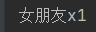## 2、 while 循环语句

while 循环语句用于「循环」执行程序，即在条件「成立」的情况下，循环执行某段程序，常用来重复执行的相同任务。

### 1）基本语法

``````while 判断条件:
条件满足时,做的事情1
条件满足时,做的事情2
...
处理条件
```12345```

``````i = 1
while i <= 5:
print(i)
i += 1
```1234```

``````i = 1
while i <= 5:
print('*' * i)
i += 1
```1234```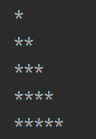### 2.2 列表

【列表 List】是Python中使用 最频繁 的数据类型，在其他语言中通常叫做 数组 ，列表用来存储 一串数据 ，数据和数据之间使用 逗号 分隔

names = [‘张三’, ‘李四’, ‘王五’]

``````di = [11, 22, 33, 44]

for di in ids:
print('my id is %%%%d' %%%% di)
```1234```### 2.3 字典

student = {‘name’: ‘小明’, ‘age’: 18, ‘id’: 1}

``````for key in student:
print('%%%%s : %%%%s' %%%% (key, student[key]))
```12```